### Home > CALC > Chapter 7 > Lesson 7.3.4 > Problem7-148

7-148.
1. Let y(t) denote the temperature (in °F) of a cup of tea at time t (in minutes). The temperature of the tea starts at 190°, while the room temperature is 70°. The tea's change in temperature is described by the equation: Homework Help ✎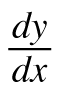= −0.1(y − 70)

1. Describe the change in temperature of the tea in relation to the room temperature.

2. How hot is the tea at any time t?

3. How hot is the tea after 10 minutes?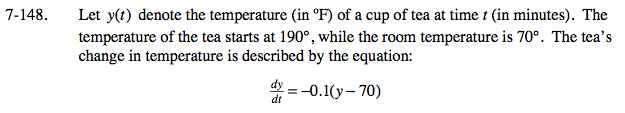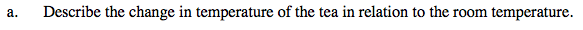As time goes on, will the tea ever become colder than the room temperature?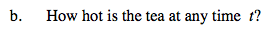Implicitly integrate to find y(t).

Find the value of 'C' by evaluating the initial condition: when t = 0, y = 190.

y = 70 + 120e−0.1t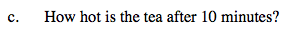y(10) = ________________